# Questions About Ellipses (7 Things You Need To Know)

Ellipses are used often in mathematics, and they also describe orbits of celestial bodies in astronomy.  An ellipse is one of the conic sections, and a circle is just a specific case of an ellipse.

So, what do you need to know about ellipses?  An ellipse is defined by 2 foci on its major axis inside the ellipse. Eccentricity tells how stretched out the ellipse is (0 for a circle). We find the center of an ellipse from its equation, its foci, or its vertices. An ellipse is not a function and has no asymptotes, but it has directrices.

Of course, we often look at an ellipse so that it is wider than it is tall (by convention).

In this article, we’ll answer some common questions about ellipses and their centers, foci, vertices, and eccentricity.  We’ll also look at some examples to make the concepts clear.

Let’s get started.

Remember that the two foci (plural of focus) are the key points that help to define an ellipse.  For a given point, we can calculate its distance from each focus.

The sum of these two distances will be a constant value for the set of points on the ellipse.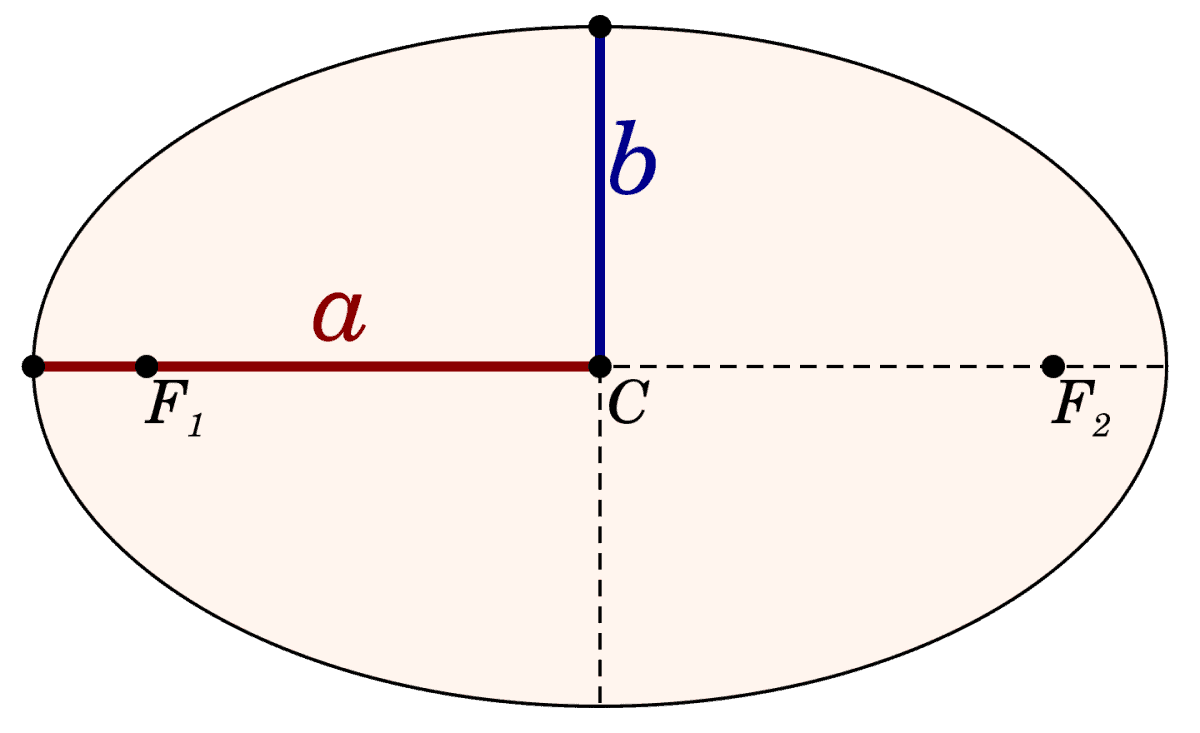The points F1 and F2 are the foci of this ellipse. Note that they lie on the major axis (which has a width of 2a). The center point C is halfway between the two foci, and lies on the minor axis (which has a height of 2b).

The center of the ellipse is the midpoint of the line segment between the two foci.

The major axis is the line through the two foci of the ellipse.

The minor axis is the line that is perpendicular to the major axis and passes through the center.

The vertices are the two points where the major axis intersects the ellipse.

If the ellipse centered at (0, 0) has width 2a and height 2b, then the equation is given by

• (x2 / a2) + (y2 / b2) = 1

where a >= b.

The foci are the points (c, 0) and (-c, 0) where c = √(a2 – b2).

### What Is The Eccentricity Of An Ellipse?

The eccentricity of an ellipse is the ratio e = c / a.  Remember that c is the distance of a focus from the center, and a is the distance of a vertex from the center (half the length of the major axis).

The eccentricity “e” of an ellipse tells us how elongated (stretched out horizontally) it is.  For 0 < e < 1, we have an ellipse.The closer the eccentricity is to 1, the more “stretched out” the ellipse becomes. When eccentricity = 0, we have a circle (a special case of an ellipse where the foci are both at the center, and the axes have the same length, equal to the diameter).

In the extreme of e = 1, we have a parabola.  Note that this means c / a = 1, or c = a (the vertex and the focus are the same point).

### Can An Ellipse Be A Circle?

An ellipse can be a circle.  In the extreme case of an eccentricity of zero (e = 0), an ellipse is a circle.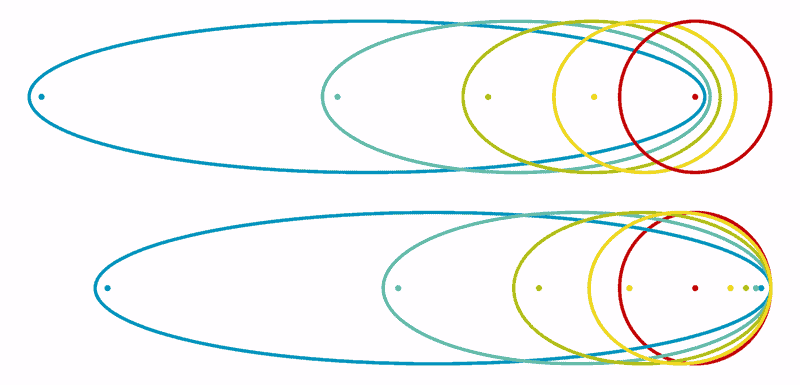As eccentricity increases (e approaches 1), an ellipse becomes more stretched out. As e approaches 0, the ellipse starts to look like a circle.

Note that this means that c / a = 0, which means c = 0.  This tells us that the foci are at the center of the ellipse, which means we have a circle.

We can also see this by looking at the formula c = √(a2 – b2).  If c= 0, then:

• c = √(a2 – b2)  [formula for c]
• 0 = √(a2 – b2)  [c = 0]
• 02 = (√(a2 – b2))2  [square both sides]
• 0 = a2 – b2
• b2 = a2
• b = a

Since a and b are lengths, they must have the same sign (positive).  So, a must equal b, and the two axes (major and minor) of the ellipse have the same length (which is also the diameter of the circle).

### How To Find The Center Of An Ellipse

There are several different ways to find the center of an ellipse, depending on what information we are given:

• from the equation of the ellipse
• from the foci of the ellipse
• from the vertices of the ellipse

Let’s start off with finding the center from the equation of the ellipse.

#### How To Find The Center Of An Ellipse From The Equation

Remember that the general equation for an ellipse centered at the origin (0, 0) is given by:

• (x2 / a2) + (y2 / b2) = 1

However, there is a more general form for an ellipse centered at (xC, yC):

• ((x- xC)2 / a2) + ((y – yC)2 / b2) = 1

Note that either form also gives us the value of a and b, which tells us the length of the major axis (2a) and the length of the minor axis (2b).

##### Example: Find The Center Of An Ellipse From An Equation

Given the ellipse with the equation

• ((x – 4)2 / 25) + ((y – 1)2 / 9) = 1

We know that the center (xC, yC) is the point (4, 1).

We also know that a2 = 25 and b2 = 9, so a = 5 and b = 3.  This means the major axis has a length of 2a = 10, and the minor axis has a length of 2b = 6.

#### How To Find The Center Of An Ellipse From The Foci

Remember that the center of an ellipse is at the midpoint of the line through the two foci.  So, the center of the ellipse will have the same y-value as the foci.

However, the x-value of the center will be halfway between the x-values of the foci.  That means that the x-value of the center is the average of the x-values of the foci.

So, if the foci are (f1, d) and (f2, d), then the center is given by:

• (xC, yC) = ((f1 + f2) / 2, d)
##### Example: Find The Center Of An Ellipse From The Foci

Given the ellipse with foci (2, 3) and (8, 3), the center is given by:

• (xC, yC) = ((f1 + f2) / 2, d)
• (xC, yC) = ((2 + 8) / 2, 3)
• (xC, yC) = (10 / 2, 3)
• (xC, yC) = (5, 3)

#### How To Find The Center Of An Ellipse From The Vertices

Remember that the center of an ellipse is at the midpoint of the line through the two vertices.  So, the center of the ellipse will have the same y-value as the vertices.

However, the x-value of the center will be halfway between the x-values of the vertices.  That means that the x-value of the center is the average of the x-values of the vertices.

So, if the vertices are (v1, d) and (v2, d), then the center is given by:

• (xC, yC) = ((v1 + v2) / 2, d)
##### Example: Find The Center Of An Ellipse From The Vertices

Given the ellipse with foci (5, 1) and (17, 1), the center is given by:

• (xC, yC) = ((v1 + v2) / 2, d)
• (xC, yC) = ((5 + 17) / 2, 1)
• (xC, yC) = (22 / 2, 1)
• (xC, yC) = (11, 1)

### Can Foci Be Outside Of The Ellipse?

Foci cannot be outside of the ellipse.  If the foci were outside of the ellipse, this would imply c > a, which means an eccentricity e = c / a that is greater than 1.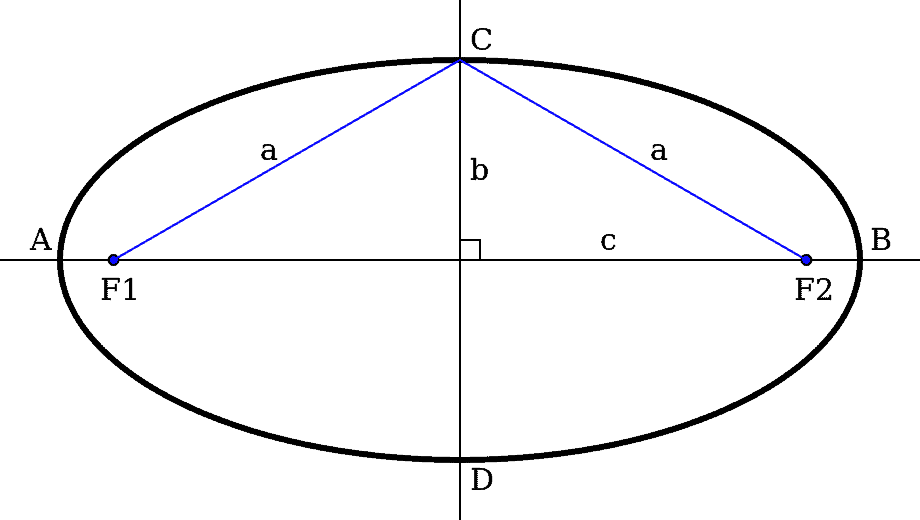The foci of an ellipse must be inside the ellipse, since the Pythagorean Theorem tells us that c is always less than a.

Also, c > a implies c2 > a2, which contradicts the equation for c in terms of a and b:

• c = √(a2 – b2)  [equation for c in terms of a and b]
• c2 = a2 – b2  [square both sides]
• c2 <= a2  [since b2 >= 0]

### Is The Graph Of An Ellipse A Function?

The graph of an ellipse cannot be a function, since it fails the vertical line test.  Specifically, the minor axis (the vertical line going through the center (xC, yC) of the ellipse) intersects the graph at the points (xC, yC + b) and (xC, yC – b).

#### Example 1: An Ellipse Failing The Vertical Line Test

Let’s say we have the ellipse

• ((x – 4)2 / 25) + ((y – 1)2 / 9) = 1

Its center is at (4, 1), and we have a = 5 and b = 3.  Then the points (4, 1 + 3) and (4, 1 – 3) are both on the minor (vertical) axis of the ellipse.

So the vertical line x = 4 intersects the ellipse at those two points: (4, 4) and 4, -2).  Thus, the graph of the ellipse fails the vertical line test, and so it is not a function, but it is a relation.

However, the top (or bottom) half of an ellipse is a function, since each input has only one output.  In fact, we can solve for y in the ellipse equation and take the positive square root to get the top half of the ellipse (the negative square root would give us the bottom half):

• ((x – xC)2 / a2) + ((y – yC)2 / b2) = 1  [standard equation of an ellipse]
• ((y – yC)2 / b2) = 1 – ((x – xC)2 / a2)  [subtract ((x- xC)2 / a2 from both sides]
• (y – yC)2 = b2(1 – ((x – xC)2 / a2))  [multiply by b2 on both sides]
• (y – yC) = √(b2(1 – ((x – xC)2 / a2)))  [take the square root of both sides]
• y = yC + √(b2(1 – ((x – xC)2 / a2)))  [add yC to both sides]

#### Example 2: A Half-Ellipse Passing The Vertical Line Test

Going back to the ellipse

• ((x – 4)2 / 25) + ((y – 1)2 / 9) = 1

we can use the formula above to solve for y to find:

• y = yC + √(b2(1 – ((x – xC)2 / a2)))
• y = 1 + √(32(1 – ((x – 4)2 / 52)))
• y = 1 + √(9(1 – ((x – 4)2 / 25)))
• y = 1 + √(9(1 – ((x2 – 8x + 16)/ 25)))
• y = 1 + 3√((1 – ((x2 – 8x + 16)/ 25)))
• y = 1 + 3√(((25 – (x2 – 8x + 16))/ 25))
• y = 1 + 3√((9 + 8x -x2)/ 25))
• y = 1 + (3/5)√(9 + 8x -x2)

This gives us the upper-half of the ellipse, shown in the graph below: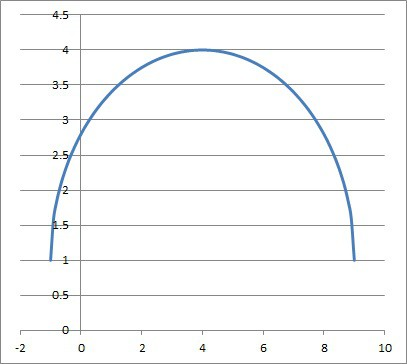The upper half of the ellipse ((x – 4)2 / 25) + ((y – 1)2 / 9) = 1.

This graph is a function, since any vertical line will intersect the half-ellipse at most once.

### Do Ellipses Have Directrix?

An ellipse has a directrix at the vertical line x = +a / e and also at the vertical line x = -a / e.  Remember that a is half the length of the major (horizontal) axis, and e is the eccentricity of the ellipse.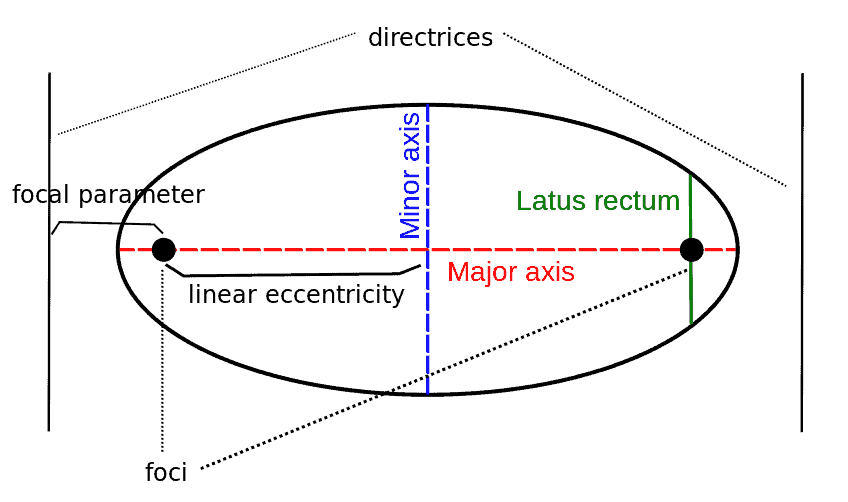An ellipse has a directrix at x = a / e and at x = -a / e.

(We can also write a / e as a2 / c, since e = c / a by definition of eccentricity).

For every point on the ellipse, the ratio between the distance to the focus and the distance to the directrix is constant.

### Do Ellipses Have Asymptotes?

Ellipses do not have asymptotes.

A vertical asymptote would require a denominator that approaches zero as x approaches some value.  However, there are no variables in the denominator of an ellipse equation.

A horizontal asymptote would require the degree of the numerator to be less than or equal to the degree of the denominator.  However, the ellipse equation has degree 2 in the numerator and degree 0 in the denominator.

## Conclusion

Now you know the answers to some common questions about ellipses.  You also know how to work with the equations and vocabulary of ellipses.